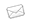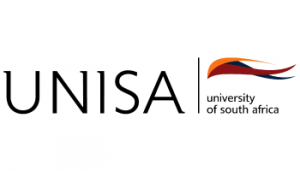# COURSE: BSC IN MATHEMATICAL AND STATISTICAL SC...N/A
###### Duration:
3 Years Full Time / Full Time / Part Time
During the programme, students will master the basic theory and some of the applications of ordinary differential equations and multivariable calculus. They will also be exposed to three of the following branches of applied mathematics: partial differential equations, numerical methods, special theory of relativity, mechanics and discrete mathematics.

Duration: 3 years part time

#### Compulsory Subjects

##### First year subjects:

• Pre-calculus Mathematics

• Calculus

• Linear algebra

• Comprehension skills for science

• End-user Computing

• Mechanics

• Applied Linear Algebra

• Mathematical Modelling

##### Second year subjects:

• Differential equations

• Calculus in higher dimensions

• Numerical methods

• Applied dynamical systems

• Computer algebra

##### Third year subjects:

• Partial differential equations

• Numerical methods

• Mechanics and the calculus of variations

• Special relativity and Riemannian geometry

• Ordinary differential equations

• Discrete mathematics: Combinatorics

Careers: mathematical modeller, statistical analyst.

Source: www.unisa.ac.za

AVAILABLE BURSARIES
No related bursaries found
RELATED COURSES
No related courses found